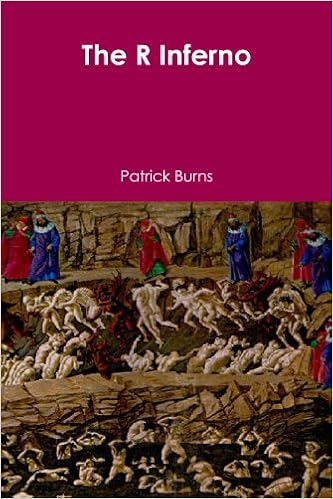## moralizing gods drive Nature rejection

Posted in Statistics with tags , , , , , , , on August 29, 2021 by xi'an

## multinomial but unique

Posted in Kids, R, Statistics with tags , , , , , , on July 16, 2021 by xi'anA quick riddle from the Riddler, where the multinomial M(n¹,n²,100-n¹-n²) probability of getting three different labels out of three possible ones out of three draws is 20%, inducing a single possible value for (n¹,n²) up to a permutation.

Since this probability is n¹n²(100-n¹-n²)/161,700, there indeed happens to be only one decomposition of 32,340 as 21 x 35 x 44. The number of possible values for the probability is actually 796, with potential large gaps between successive values of n¹n²(100-n¹-n²) as shown by the above picture.

## almost reversed 2-lag Markov chain

Posted in Kids, R, Statistics with tags , , , , on July 7, 2021 by xi'an

Another simple riddle from the Riddler: take a binary sequence and associate to this sequence a score vector made of the numbers of consecutive ones from each position. If the sequence is ten step long and there are 3 ones located at random, what is the expected total score? (The original story is much more complex and involves as often strange sports!)

Adding two zeroes at time 11 and 12, this is quite simple to code, e.g.

```f=0*(1:10) #frequencies
for(v in 1:1e6){
r=0*f#reward
s=sample(1:10,3)
for(t in s)r[t]=1+((t+1)%in%s)*(1+((t+2)%in%s))
f[sum(r)]=f[sum(r)]+1}
f=f/1e6
```

and the outcome recovers the feature that the only possible scores are 1+1+1=3 (all ones separated), 1+1+2=4 (two ones contiguous),  and 1+2+3=6 (all ones contiguous). With respective frequencies 56/120, 56/120, and 8/120. With 120 being the number of possible locations of the 3 ones.

## breaking sticks of various length

Posted in Kids, pictures, R with tags , , , , on July 6, 2021 by xi'anA riddle from the Riddler with a variation on the theme of breaking sticks: Given a stick of length L, what is the optimal manner to break said stick to achieve a maximal product of the individual lengths? While the pen & paper resolution is a one-line back-of-the-envelope calculation, with an impact of the length L, obviously,  a quick R code leads to an approximate solution:

```mw=function(k=2,l=10,T=1e6){
a=matrix(runif(T*k),k)
for(i in 1:T)F=max(F,prod(l*a[,i]/sum(a[,i])))
return(F)}
```

with increasing inaccuracy when L grows, obviously.

## inf R ! [book review]

Posted in Books, R, Travel with tags , , , , , , , , , , , on June 10, 2021 by xi'anThanks to my answering a (basic) question on X validated involving an R code, R mistakes and some misunderstanding about Bayesian hierarchical modelling, I got pointed out to Patrick Burns’ The R inferno. This is not a recent book as the second edition is of 2012, with a 2011 version still available on-line. Which is the version I read. As hinted by the cover, the book plays on Dante’s Inferno and each chapter is associated with a circle of Hell… Including drawings by Botticelli. The style is thus most enjoyable and sometimes hilarious. Like hell!

The first circle (reserved for virtuous pagans) is about treating integral reals as if they were integers, the second circle (attributed to gluttons, although Dante’s is for the lustful) is about allocating more space along the way, as in the question I answered and in most of my students’ codes! The third circle (allocated here to blasphemous sinners, destined for Dante’s seven circle, when Dante’s third circle is to the gluttons) points out the consequences of not vectorising, with for instance the impressive capacities of the ifelse() function [exploited to the max in R codecolfing!].  And the fourth circle (made for the lustfuls rather than Dante’s avaricious and prodigals) is a short warning about the opposite over-vectorising. Circle five (destined for the treasoners, and not Dante’s wrathfuls) pushes for and advises about writing R functions. Circle six recovers Dante’s classification, welcoming (!) heretics, and prohibiting global assignments, in another short chapter. Circle seven (alloted to the simoniacs—who should be sharing the eight circle with many other sinners—rather than the violents as in Dante’s seventh) discusses object attributes, with the distinction between S3 and S4 methods somewhat lost on me. Circle eight (targeting the fraudulents, as in Dante’s original) is massive as it covers “a large number of ghosts, chimeras and devils”, a collection of difficulties and dangers and freak occurences, with the initial warning that “It is a sin to assume that code does what is intended”. A lot of these came as surprises to me and I was rarely able to spot the difficulty without the guidance of the book. Plenty to learn from these examples and counter-examples. Reaching Circle nine (where live (!) the thieves, rather than Dante’s traitors). A “special place for those who feel compelled to drag the rest of us into hell.” Discussing the proper ways to get help on fori. Like Stack Exchange. Concluding with the tongue-in-cheek comment that “there seems to be positive correlation between a person’s level of annoyance at [being asked several times the same question] and ability to answer questions.” This being a hidden test, right?!, as the correlation should be negative.